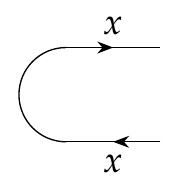Another question I feel a bit ashamed to ask, but better late than never: Is it correct thatcould be drawn as

![Imgur](https://i.imgur.com/ZugI2bol.png)

-----

Then we have a \$$X \times X^\ast \$$ -wire leaving from the right and a \$$1 \$$-wire coming in from the left. We usually do not draw the \$$1 \$$-wire, because (a) when it is the only wire coming in, it is uninteresting and when (b) there are other wires coming in, the resulting product is isomorphic to the product without the wire. (I.e. assume a \$$1 \$$-wire, a \$$Y \$$-wire and a \$$Z \$$-wire are leading into the box. The resulting product is \$$1 \times Y \times Z \$$ (in any order). This is isomorphic to \$$Y \times Z \$$).